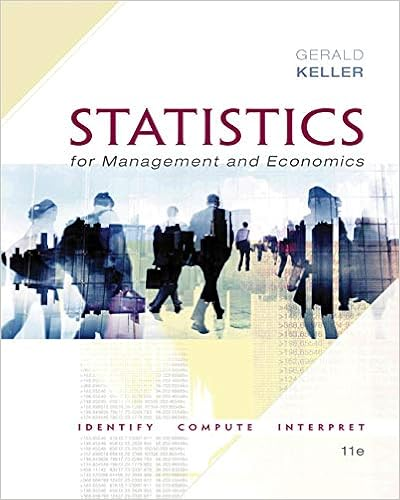# Arranged in order the n10 scores 39 43 51 53 62 63 73

• nolio93
• 28
• 100% (1) 1 out of 1 people found this document helpful

This preview shows page 1 - 3 out of 28 pages.

##### We have textbook solutions for you!
The document you are viewing contains questions related to this textbook.The document you are viewing contains questions related to this textbook.
Chapter 15 / Exercise 15.46
Statistics for Management and Economics + XLSTAT Bind-in
KellerExpert Verified
5. Arranged in order, the n=10 scores are: 34 36 39 43 51 53 62 63 73 79
##### We have textbook solutions for you!
The document you are viewing contains questions related to this textbook.The document you are viewing contains questions related to this textbook.
Chapter 15 / Exercise 15.46
Statistics for Management and Economics + XLSTAT Bind-in
KellerExpert Verified
Measures of Center SECTION 3.2 45 language, since the dictionary does not claim to contain every word. NOTE: The exercise also asks whether the estimate for the total is “accurate.” This is a relative term. A change of 1.0 in the estimate for the mean produces a change of 1459 in the estimate for the total. While a change of 1459 is only a change of 1459/77765 = 1.9%, this illustrates that accuracy in terms of absolute numbers is sometimes hard to attain when estimating totals. 6. Arranged in order, the n=6 scores are: 431 546 612 649 774 1210a. x = (Σx)/n = (4222)/6 = 703.7 hic c. M = (none) b. x±= (612 + 649)/2 = 630.5 hic d. m.r. = (431 + 1210)/2 = 820.5 hic No. Even though all four measures of center fall within with accepted guidelines, all the individual values do not. Since one result exceeds the guidelines, it is clear that allchild booster seats do not meet the requirement. NOTE: Even for a very large sample (e.g., n=10,000), if one sample value exceeds the guidelines then it is clear that all the seats in the population do not meet the requirement. For the given sample of size n=6, a sample failure rate of 1/6 = 17% suggests a population failure rate too large to ignore. For a sample of size n=10,000, a sample failure rate of 1/10,000 = 0.01% would likely be acceptable – even though allseats still do not meet the requirement. 7. Arranged in order, the n=5 scores are: 4277 4911 6374 7448 9051a. x = (Σx)/n = (32061)/5 = \$6412.2 c. M = (none) b. x±= \$6374 d. m.r. = (4277 + 9051)/2 = \$6664.0 No. Even though the sample values cover a fairly wide range, the measures of center do not differ very much. 8. Arranged in order, the n=12 scores are: 664 693 698 714 751 753 779 789 802 818 834 836 a. x = (Σx)/n = (9131)/12 = 760.9 points c. M = (none) b. x±= (753 + 779)/2 = 766.0 points d. m.r. = (664 + 836)/2 = 750.0 points No. The sample mean of 760.9 appears to be significantly higher than the reported mean, and 11 of the 12 values are higher than the reported mean. The sample FICO scores do not appear to be consistent with the reported mean. 9. Arranged in order, the n=10 scores are: 8.4 9.6 10 12 13 15 27 35 36 38
•••